When building a Machine Learning model, we first choose a machine learning algorithm, then choose hyperparameters for the model, then fit the model to the training data, and then we use the model to predict labels for new data.

But how do we know if the predictions made by our model are valid and highly accurate? This is where we come across Model Validation.

#### What is Model Validation?

In machine learning, after we have chosen a model and its hyperparameters, model validation gives us an estimate, how effective it is, by applying it to some of the training data and comparing the prediction to the known value.

#### Python Implementation

Let us begin by importing the required python libraries and loading our dataset. We will be using the iris dataset to evaluate the performance of the model.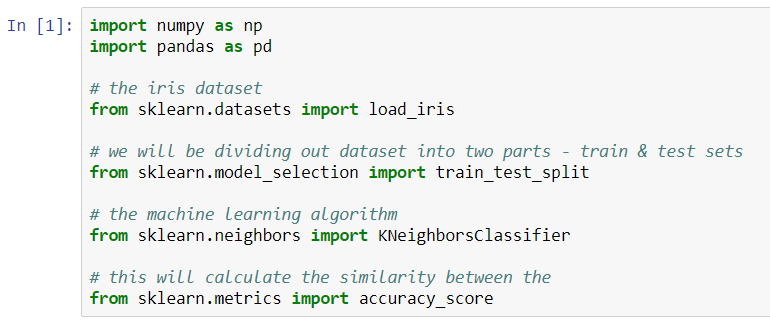Loading our dataset. The X contains the feature vectors and y contains the target values.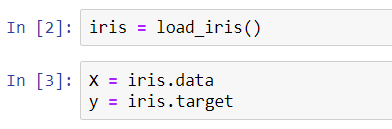We will divide our dataset into two parts. We will use the first part to train our model and the second part to test the accuracy of the model.

We can see that we have split the data into two equal halves (50% each).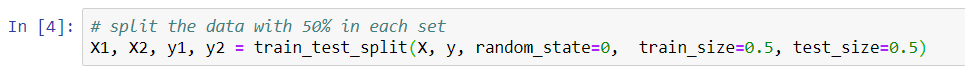We will use the K-Nearest Neighbors machine learning algorithm for building a model.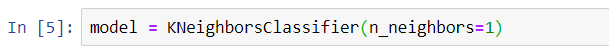Let us train our model using the training dataset we have created.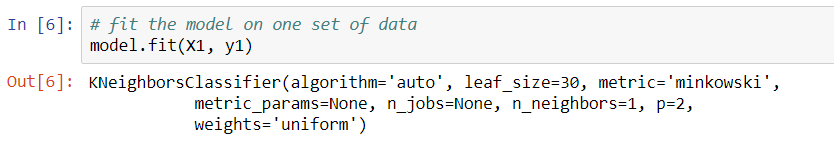Let us evaluate our model using the testing dataset we have created.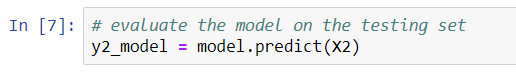Let us now calculate the accuracy of our model. Sklearn provides several functions for doing that. We are going to use the accuracy_score() function to fulfill our purpose.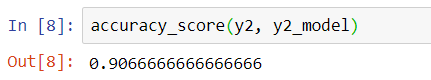In simple terms, the above function calculates how much similar is the observed values to the calculated (predicted) values.

The above model has an accuracy score of 90.66%. Though, we created a very simple model just to understand what model validation is.

If in any case, this accuracy score is low, we will change the value of the hyperparameters (n_neighbors in the above example) used in the model, and retest it till we get a decent accuracy score.